## Search This Blog

### 351 versus 2048

We've already discussed a justification for labelling all possible musical scales (based on a 12 tone chromatic, well-tempered octave of pitch classes) with a number in the range 2048 to 4095. This is a system of keyless scales, in that each scale contains (at the very least) one note identified as note zero - and usually quite a few more. This distinguished note is otherwise known as the tonic note or the scale root, where we don't care which particular note (in the world of real, played, music) it actually is. Each 'abstract' scale so identified could have twelve 'applications' where that tonic or root note takes on a value of C, or C#, or D, etc all the way up to B, thus bringing us into the territory of musical keys.

But we don't need to go there. There's still much that can be said about scales without getting all concreted up. For instance, how many scales are there with one note? How many with two? With three, four, etc all the way up to the full twelve? When divided up this way, we can see the following twelve polygonal scale systems:

 1 unigon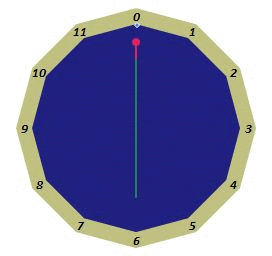1 dodecagon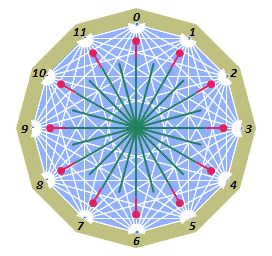11 digons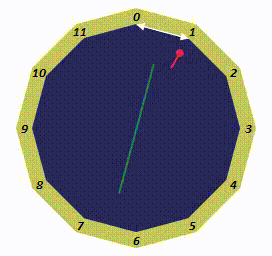11 undecagons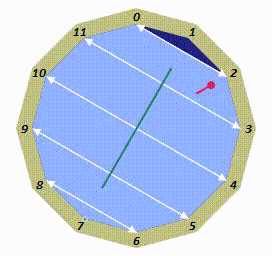55 trigons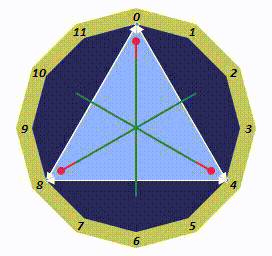55 decagons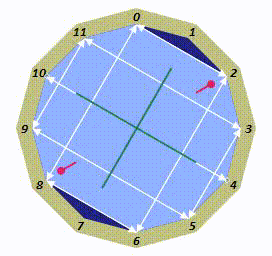165 tetragons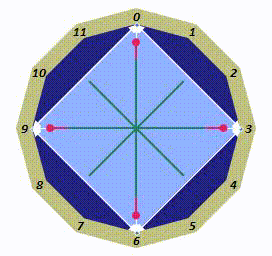165 nonagons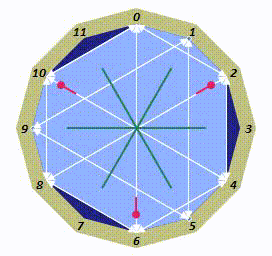330 pentagons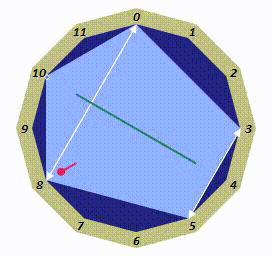330 octagons462 hexagons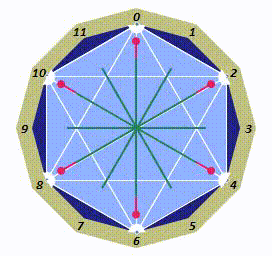462 heptagons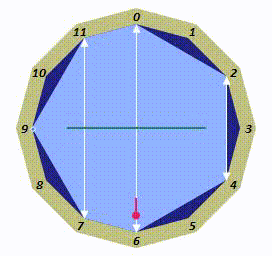It doesn't take much to figure out that there is only one scale with one note in it - bearing in mind that we are not bothered about what that note is (i.e. G, C#. A, whatever). It's also obvious that there's only one scale with twelve notes in it.

As for the rest, it's just a case of counting all twelve cardinality subsets of twelve objects (excluding the thirteenth empty set, which is of no interest to us). The usual binomial expansion does the enumeration for us - 2048 = 211 = (1 + 1)11 = (1 + x)11 where x = 1. I.e. the coefficients of the powers of x in the expansion of (1 + x)11 do the trick, viz:

1 + 11x + 55x2 + 165x3 + 330x4 + 462x5 + 462x6 + 330x7 + 165x8 + 55x9 + 11x10 + x11

One might add up those coefficients to see that they total 2048 but all you need do is set x = 1 and remember how we got here. But do go ahead if you wish! That this is telling us that there are eleven scales with two notes should fit with expectations since - having chosen the root note (and this must be the one at zero, or twelve o'clock on a clock face) - only eleven places remain for the only other note of the scale to occupy. And here they all are: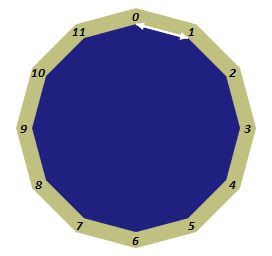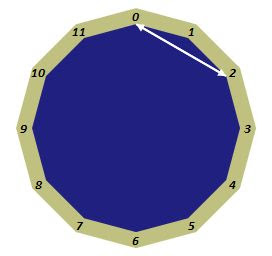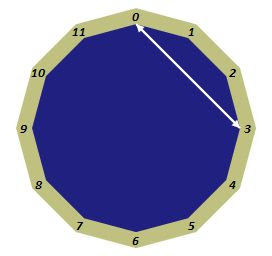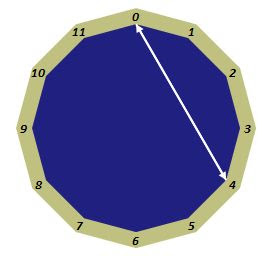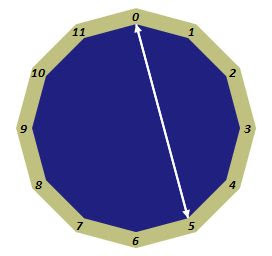The eleven possible two-note scales as 2-gons on a clock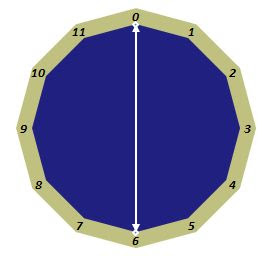Note the symmetries of the first and last five scales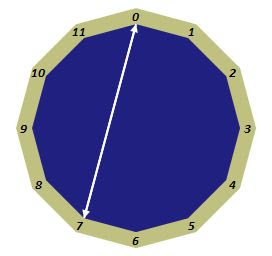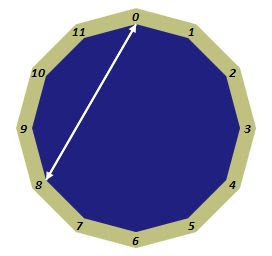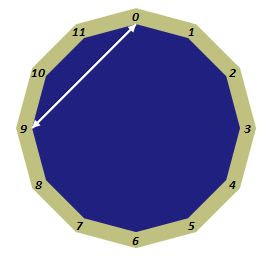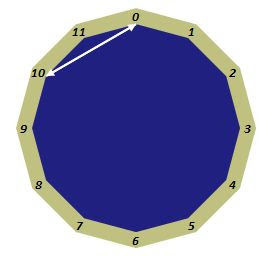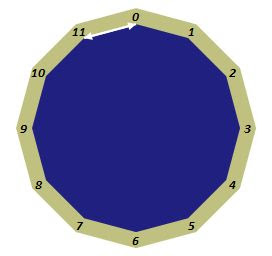This also - and symmetrically - helps us identify what must perforce be the eleven scales containing eleven notes because there are only eleven places that we can omit a note from the full twelve note chromatic scale (we must keep that sacred zero position occupied).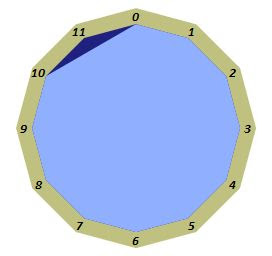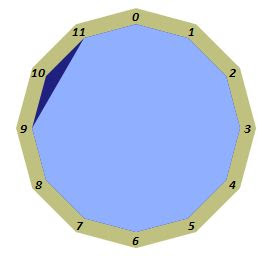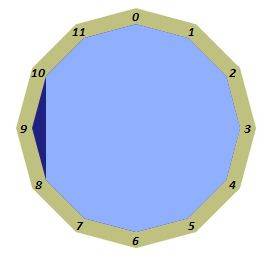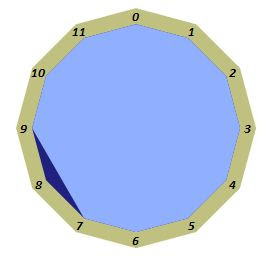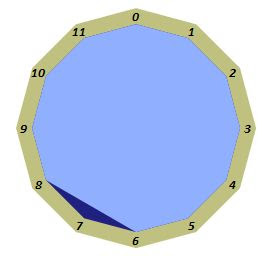The eleven possible eleven-note scales as undecagons on a clockNote that they are all basically the same scale, rotated eleven times around the clock.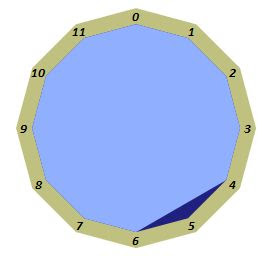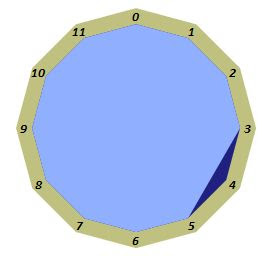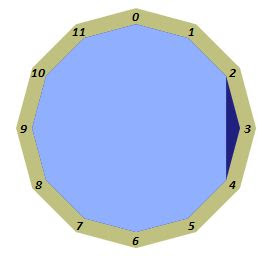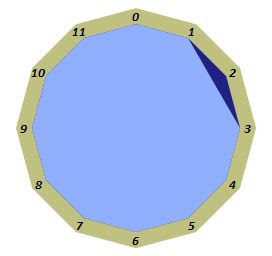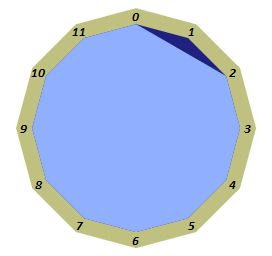That there are 55 three (and, symmetrically, ten) note scales is less obvious but if you know how to enumerate combinations then you're already sold on the idea. If you don't, then there are oodles of places where you can learn about counting combinations.

## But how many are there really?

From the above illustrations we can see that although the binomial expansion of (1 + x)11 tells us we have 11 choices for two note scales (11 places to put the first and only extra note to accompany the fixed one at twelve o'clock), 5 of them are 'the same' - in that they're just reflections (or rotations - the 'semitone' scale from twelve to one could be reflected in the twelve-six axis, or just as validly rotated eleven 'hours' to the eleven to twelve scale) of each other (major-minor, if you will) and that the 6th (the twelve o'clock - six o'clock scale) is out there on its own. We thus count those 11 scales as 6 (5 pairs plus a singleton). Similarly, as all 11 eleven note scales are simple rotations of each other, there's only 1 eleven note scale (with eleven 'modes', each starting on a different tonic note, if you will).

Similarly, the 55 places you can locate the two extra, non-tonic, notes in three note scales can be reduced to representations as 19 different triangles. Of these, 1 is the equilateral triangle sitting at twelve-four-eight o'clock. Rotating this does nothing so there's only 1 scale there (the three note 'augmented' scale). Further symmetric (along the vertical twelve-six axis) scales are 4 isosceles triangles sitting on the other 'hours' twelve-(one-eleven, two-ten, three-nine, five-seven). Each of these can be rotated without ending up as the identical scale. So far, that's 1 × 1 + 4 × 3 = 13 accounted for from the 55. What remain are a further 14 as 7 pairs of scalene triangles, each with 3 rotatable variants of the same basic shape. That last 3 × 14 = 42 brings us up to 55. Here are some pictures to show you what's going on:

 The one and only augmented scale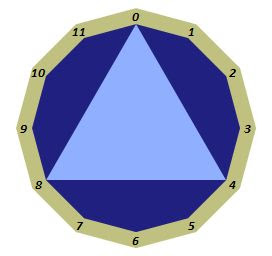Four 'isosceles' scales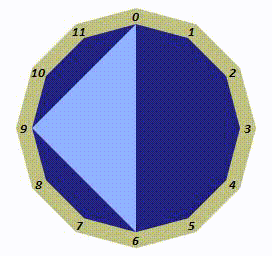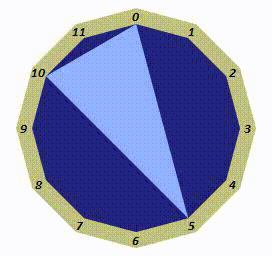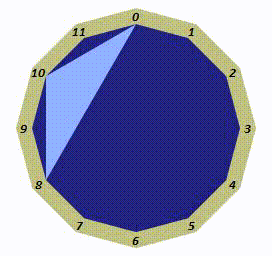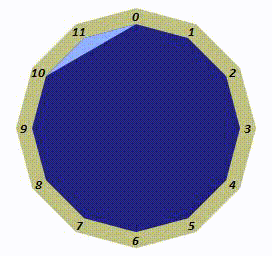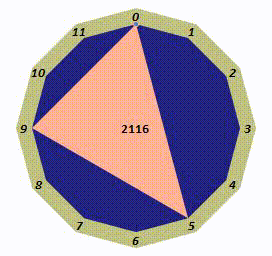Seven 'scalene' pairs of antisymmetric triplets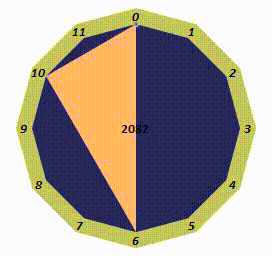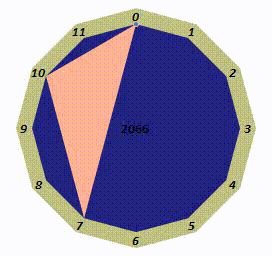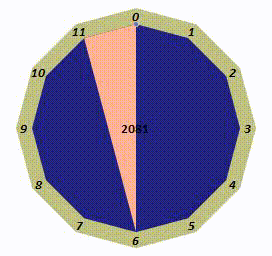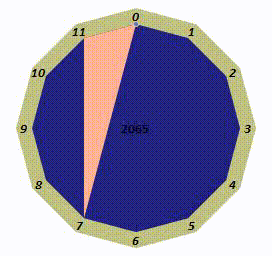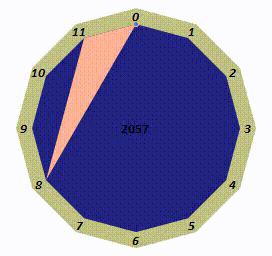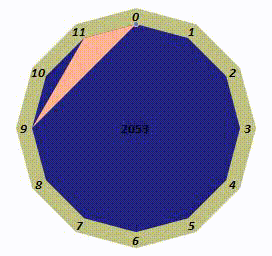That's 1 + 4 + 2 ×'7 = 19 folks!

The 55 ten note scales have similar symmetries. One group is symmetric in two axes at right angles to each other and thus has only 5 distinct orientations within the clock. Another five groups have a single axis of symmetry and - having 10 orientations within the clock face, account for the other 50:

 1 doubly symmetric 10 note scale with its 5 modes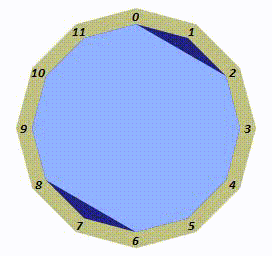5 singly symmetric scales, 10 rotations (aka modes) each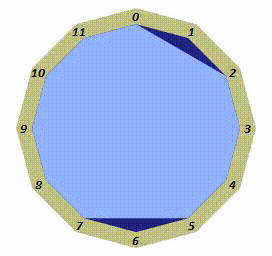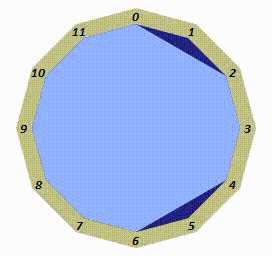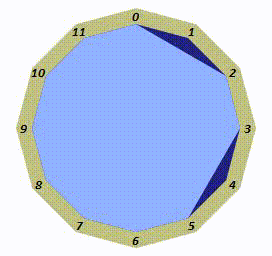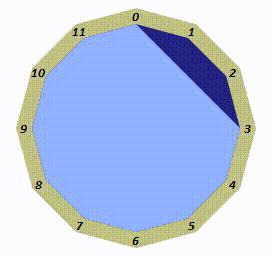We can perform similar reductions - via symmetry and rotation - on the other scales and the totals given by the 'raw' binomial expansion reduce as follows

Number of notes in scale
123456789101112total
11155165330462 46233016555111 2048
1619436680 664319611 351

So that's why there are only, really, 351 scales. We count the rotationally equivalent polygons in the exact same way that we count the seven distinct modes of the western diatonic (heptatonic) scale. The heptagon's shape is constant, but each vertex of the heptagon gets a turn (quite literally, as it happens) at being the tonic note of the scale at that coveted twelve o'clock position.

But it's not quite over yet.